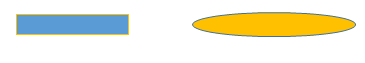# SMHS BigDataBigSci SEM

## Model-based Analytics - Structural Equation Modeling (SEM)

SEM allow re-parameterization of random-effects to specify latent variables that may affect measures at different time points using structural equations. SEM show variables having predictive (possibly causal) effects on other variables (denoted by arrows) where coefficients index the strength and direction of predictive relations. SEM does not offer much more than what classical regression methods do, but it does allow simultaneous estimation of multiple equations modeling complementary relations.

SEM is a general multivariate statistical analysis technique that can be used for causal modeling/inference, path analysis, confirmatory factor analysis (CFA), covariance structure modeling, and correlation structure modeling.

• It allows testing models with multiple dependent variables
• Provides mechanisms for modeling mediating variables
• Enables modeling of error terms
• acilitates modeling of challenging data (longitudinal with auto-correlated errors, multi-level data, non-normal data, incomplete data)

SEM allows separation of observed and latent variables. Other standard statistical procedures may be viewed as special cases of SEM, where statistical significance less important, than in other techniques, and covariances are the core of structural equation models.

### Definitions

• The disturbance, D, is the variance in Y unexplained by a variable X that is assumed to affect Y.
```X    →    Y   ←     D
```
• Measurement error, E, is the variance in X unexplained by A, where X is an observed variable that is presumed to measure a latent variable, A.
```A    →    X   ←     E
```
• Categorical variables in a model are exogenous (independent) or endogenous (dependent).

### Notation

• In SEM observed (or manifest) indicators are represented by squares/rectangles whereas latent variables (or factors) represented by circles/ovals.• Relations: Direct effects (→), Reciprocal effects (↔ or ⇆), and Correlation or covariance (⤻ or ⤺) all have different appearance in SEM models.

### Model Components

The measurement part of SEM model deals with the latent variables and their indicators. A pure measurement model is a confirmatory factor analysis (CFA) model with unmeasured covariance (bidirectional arrows) between each possible pair of latent variables. There are straight arrows from the latent variables to their respective indicators and straight arrows from the error and disturbance terms to their respective variables, but no direct effects (straight arrows) connecting the latent variables. The measurement model is evaluated using goodness of fit measures (Chi-Square test, BIC, AIC, etc.) Validation of the measurement model is always first.

Then we proceed to the structural model (including a set of exogenous and endogenous variables together with the direct effects (straight arrows) connecting them along with the disturbance and error terms for these variables that reflect the effects of unmeasured variables not in the model).